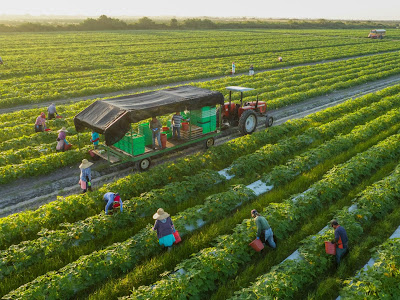--># Crop Combination Theory by Rafiullah

### Crop Combination by Rafiullah

Looking at the inherent weakness of Weaver’s method which tends to include all or most of the crops in the series by which the resultant combination become over-generalized, Rafiullah (1956) developed a new deviation method in his work New Approach to the Functional Classification of Towns. Raffiullah,s combination method is known as maximum positive deviation method. From the calculation, maximum value is taken into consideration.
The technique devised by Rafiullah may be expressed as follows:
where d is the deviation, Dp is the positive difference and Dn is the negative difference from the median value of the theoretical curve value of the combination, and N is the number of functions (crops) in the combination.
Since it is the relative rank of the value of deviation which is needed, the under root sign may be ignored to save labori­ous calculations and the formula may be used in the following form:
To illustrate Rafiullah’s maximum positive deviation method an illustration can be given from the Basti district of Uttar Pradesh, in which rice occupies 54 per cent, wheat 23 per cent, barley 9 per cent and sugarcane 5 per cent of the total cropped area.Since the variance of the 2-crop combination (209.25) is the maximum positive deviation, the district is designated with the 2- crop combination, i.e., rice and wheat (RW).

In the maximum positive deviation method, unlike the standard deviation method, the differences of actual values are calculated from the middle value of the theoretical standard and thus this method also gives the desired critical combination. An application of the maximum positive deviation method to the agricultural data of Uttar Pradesh results in the recognition of 6-crop combination. The combinations are mapped in Figure 7.7.
A comparison of Figure 7.5 and Figure 7.7 reveals that the later is identical in 16 districts, lower in 32 districts and higher in four dis­tricts. In other words, the maximum positive deviation method in­cludes lesser number of crops in combination and thus avoids the in­clusion of insignificant crops from the combination.
The statistical technique advocated by Raffiullah is more accu­rate, objective, and scientific, and therefore quite popular for the de­lineation of crop combination regions. This technique has the capac­ity to handle the highly diversified cropping structures. In general, the crop combinations demarcated on the basis of statistical tech­niques provide a sound base for agricultural planning and develop­ment.